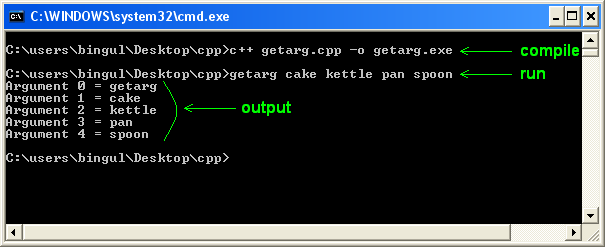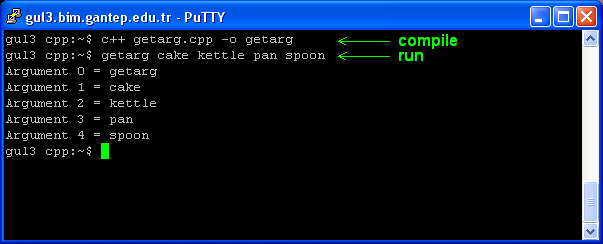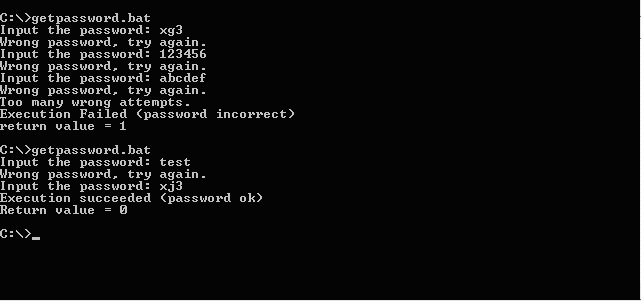http://cpp.gantep.edu.tr
C++ resources. Faculty of Engineering, University of Gaziantep
MAIN  -  Tutorials  -  Howtos  -  nmPrimer  -  Misc  -  Links  -  Contacts

Complete: ###################- (95%)

Passing parameters on execution of a program.
2.   The return values of the main() Function

Command arguments allow a program to take data from the execution command line. This is done by passing two arguments to the main() function as follows:
```  int main(int argc, char **argv)
{
.
.
.
}
```
where:
• argc   is number of command line parameters
• argv   is an array of character pointers (strings).
This argument to main the command line parameters include the entire parsed command line (including the program name).
Note that, even you give the parameters as a numerical literals, they are assumed to be string in the program. In order to convert these character strings (taken from command line) to a numerical values, the following functions defined in <cstdlib> can be used:
• int atoi(string) converts a string to integer.
• int atol(string) converts a string to long integer.
• double atof(string) converts a string to double.

Some examples are given below; they are compiled both under Windows and Linux operating systems.

getarg.cpp: Command-line arguments are listed.
 ``` 1: 2: 3: 4: 5: 6: 7: 8: 9: 10: 11: ``` ```// Outputs each argument separately #include using namespace std; int main(int argc, char *argv[]) { for(int i=0; i

Compile and run under Windows MS-DOS command prompt. (click here for a howto compile a program in the command line under Windows).Compile and run under Linux operating system. (click here for a howto compile a program in the command line under Linux).More examples:

 ``` 1: 2: 3: 4: 5: 6: 7: 8: 9: 10: 11: 12: 13: 14: 15: 16: 17: 18: 19: 20: 21: 22: 23: 24: 25: 26: ``` ```// Adds two integer numbers that are input from keyboard. #include #include int main(int argc, char *argv[]) { using namespace std; if(argc == 1) { cout << "Usage: add " << endl; return 0; } if(argc != 3) { cout << "Wrong number of parameters." << endl; return 1; } // get the parameters and convert them into integers int a = atoi(argv); // first param. int b = atoi(argv); // second param. cout << "The sum is " << a + b << endl; return 0; }``` ```[compile] \$ c++ add.cpp -o add [run] \$ add Usage: add \$ add 3 Wrong number of parameters. \$ add 3 4 5 Wrong number of parameters. \$ add 3 5 The sum is 8 ```

 ``` 1: 2: 3: 4: 5: 6: 7: 8: 9: 10: 11: 12: 13: 14: 15: 16: 17: 18: 19: 20: 21: 22: 23: ``` ```// Adds all values given in the command line. #include #include int main(int argc, char *argv[]) { using namespace std; if(argc==1 ) { cout << "Usage: addall ..." << endl; return 0; } double sum = 0.0; // get the parameters and convert them into real for(int p=0; p < argc; p++) sum += atof(argv[p]); cout << "The sum is " << sum << endl; return 0; }``` ```[compile] \$ g++ addall.cpp -o addall [run] \$ addall Usage: addall number1 number2 ... \$ addall 1.2 4.3 The sum is 5.5 \$ addall 11 22 The sum is 33 \$ addall 11 22 33 The sum is 66 \$ addall 11 22 33 44 The sum is 110 ```

calc.cpp: A Simple calculator performing 5 basic operations.
 ``` 1: 2: 3: 4: 5: 6: 7: 8: 9: 10: 11: 12: 13: 14: 15: 16: 17: 18: 19: 20: 21: 22: 23: 24: 25: 26: 27: 28: 29: 30: 31: 32: 33: 34: 35: 36: 37: 38: 39: 40: 41: 42: 43: 44: 45: 46: ``` ```// Basic command-line calculator // This program performs five basic operations on two integers #include #include #include using namespace std; int main(int argc, char **argv) { if(argc ==1 ) { cout << "Usage: calc ..." << endl; return 0; } if(argc < 4) { cout << "Missing parameter. " << endl; return 1; } if(argc > 4) { cout << "Too many parameters." << endl; return 2; } double x = atof(argv); // 1st par. number1 char op = argv; // 2nd par. operator double y = atof(argv); // 3rd par. number2 double z; switch(op) { case '+': z = x + y; break; case '-': z = x - y; break; case 'x': z = x * y; break; case '/': z = x / y; break; case '^': z = pow(x, y); break; default : z = 0.0; cout << "Invalid operator: " << op << endl; return 3; } cout << x << op << y << " = " << z << endl; return 0; }``` ```[compile] \$ c++ calc.cpp -o calc [run] \$ ./calc Usage: calc ... \$ ./calc 2 + 4 2+4 = 6 \$ ./calc 2 - 4 2-4 = -2 \$ ./calc 2 x 4 2x4 = 8 \$ ./calc 2 / 4 2/4 = 0.5 \$ ./calc 2 ^ 4 2^4 = 16 \$ ./calc 2 ! 4 Invalid operator: ! ```

• mycp.cpp : a basic form of the cp command in linux.
• mywc.cpp : a basic form of the wc command in linux.

2.   The return values of the main() Function

When a program terminates, the main() function returns a value. All ANSI-compliant programs declare main() to return an int. This value communicates to the operating system (OS), or the user, the status of the program. A zero usually indicates that the program exited normally, any other value represents an error code.

```  int main()
{
.
.
return 0;
}
```

If the return statement is omitted, a program will return 0. Defining main() with a type other than int (including void) will result in an error message from the compiler such as error: '::main' must return 'int' though this may be permitted in older compilers.

Returning an integer enables the program to communicate status information to other programs or scripts that invoke the executable file. Consider the following password program.

 ``` 1: 2: 3: 4: 5: 6: 7: 8: 9: 10: 11: 12: 13: 14: 15: 16: 17: 18: 19: 20: 21: 22: 23: 24: 25: 26: 27: 28: 29: 30: ``` ```// A basic password program #include using namespace std; int main() { string passwd = "xj3", p; int chance = 3; do { cout << "Input the password: "; cin >> p; if(p != passwd) cout << "Wrong password, try again.\n"; else break; chance--; } while(chance>0); // this return value will be used by another application if (chance) return 0; else { cout << "Too many wrong attempts.\n"; return 1; } }``` ```[run 1] Input the password: xg3 Wrong password, try again. Input the password: xc3 Wrong password, try again. Input the password: xd3 Too many wrong attempts. [The program exits with the return value 1] [run 2] Input the password: xg3 Wrong password, try again. Input the password: xj3 [The program exits with the return value 0] ```
 ```@echo off REM getpassword.bat password @if "%ERRORLEVEL%" == "0" goto good :fail echo Execution Failed (password incorrect) echo return value = %ERRORLEVEL% goto end :good echo Execution succeeded (password ok) echo Return value = %ERRORLEVEL% REM you can put another application(s) here... goto end :end ``````#!/bin/sh # getpassword.sh # run the c++ program ./password # copy the return value rv=\$? if [ \$rv -eq 0 ] then echo 'Execution succeeded (password ok)' echo "Return value = \$rv" # you can put another application(s) here... else echo 'Execution Failed (password incorrect)' echo Return value = \$rv fi ```